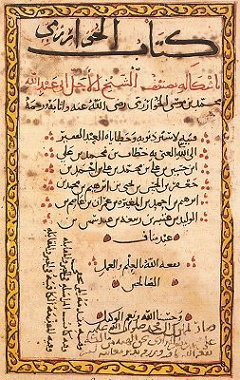# Introductory Algebra

Algebra is the branch of mathematics where variables (letters) represent numbers.

For example, we use algebra to find the perimeter of a square when we substitute the side length into the rule P = S × 4.

## Example of Algebra Words

 3a + 4y – 2x + 5
• Variable - the letters representing numbers (e.g. a, y, x)
• Coefficient - the number multiplied by the variable (e.g. 3, 4, –2)
• Constant - the number not associated with variables and that stands alone (e.g. 5)
• Term - the parts of the algebraic expression or "phrase" (e.g. 3a, 4y, –2x, 5)
• Expression - the algebraic "phrase" (e.g. 3a + 4y – 2x + 5)

## Did You Know That...?The word "algebra" comes from the Arabic language. The Arabic word "al-jebr" means "reunion of broken parts".

## Question

An expression is 5x – 3b + 10 – 4c. State the variables, coefficients, constant and terms.